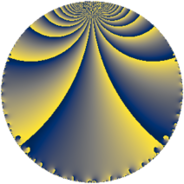Properties

 Label 35.3.iLevel $35$ Weight $3$ Character orbit 35.i Rep. character $\chi_{35}(19,\cdot)$ Character field $\Q(\zeta_{6})$ Dimension $12$ Newform subspaces $1$ Sturm bound $12$ Trace bound $0$

Related objects

Defining parameters

 Level: $$N$$ $$=$$ $$35 = 5 \cdot 7$$ Weight: $$k$$ $$=$$ $$3$$ Character orbit: $$[\chi]$$ $$=$$ 35.i (of order $$6$$ and degree $$2$$) Character conductor: $$\operatorname{cond}(\chi)$$ $$=$$ $$35$$ Character field: $$\Q(\zeta_{6})$$ Newform subspaces: $$1$$ Sturm bound: $$12$$ Trace bound: $$0$$

Dimensions

The following table gives the dimensions of various subspaces of $$M_{3}(35, [\chi])$$.

Total New Old
Modular forms 20 20 0
Cusp forms 12 12 0
Eisenstein series 8 8 0

Trace form

 $$12 q + 6 q^{4} - 18 q^{9} + O(q^{10})$$ $$12 q + 6 q^{4} - 18 q^{9} - 54 q^{10} + 6 q^{11} - 66 q^{14} + 48 q^{15} - 6 q^{16} + 18 q^{19} + 12 q^{21} + 216 q^{24} + 18 q^{25} + 18 q^{26} + 48 q^{30} - 108 q^{31} + 222 q^{35} - 204 q^{36} - 240 q^{39} - 162 q^{40} - 42 q^{44} - 216 q^{45} + 114 q^{46} - 324 q^{49} - 192 q^{50} + 180 q^{51} + 252 q^{54} + 336 q^{56} + 396 q^{59} + 384 q^{60} - 108 q^{61} + 372 q^{64} - 54 q^{65} - 108 q^{66} + 300 q^{70} + 192 q^{71} - 594 q^{74} - 216 q^{75} - 192 q^{79} - 504 q^{80} + 294 q^{81} - 1200 q^{84} - 192 q^{85} - 384 q^{86} + 684 q^{89} - 72 q^{91} + 990 q^{94} + 288 q^{95} - 540 q^{96} + 396 q^{99} + O(q^{100})$$

Decomposition of $$S_{3}^{\mathrm{new}}(35, [\chi])$$ into newform subspaces

Label Dim. $$A$$ Field CM Traces $q$-expansion
$a_{2}$ $a_{3}$ $a_{5}$ $a_{7}$
35.3.i.a $12$ $0.954$ $$\mathbb{Q}[x]/(x^{12} - \cdots)$$ None $$0$$ $$0$$ $$0$$ $$0$$ $$q+\beta _{1}q^{2}+(-\beta _{1}-\beta _{11})q^{3}+(1+\beta _{3}+\cdots)q^{4}+\cdots$$